• 本教程分享：《matlab对数函数》，MATLAB 中如何输入 对数函数方法/步骤1、自然数对数 log(x)我们在MATLAB主窗口中输入a1=log(2.7183)，回车，我们可以看到a1近似为1，e约等于2.7183,2、以2为底数的对数 log2(x)我们...
本教程分享：《matlab对数函数》，MATLAB 中如何输入 对数函数方法/步骤1、自然数对数 log(x)我们在MATLAB主窗口中输入a1=log(2.7183)，回车，我们可以看到a1近似为1，e约等于2.7183,2、以2为底数的对数 log2(x)我们在MATLAB主窗口中输入a2=log2(4) ，回车，可以看到结果a2=23、以10为底数的对数 log10(x)我们在MATLAB主窗口中输入a3=log10(10) ，回车，可以看到结果a3=14、其他底数对数logM(N)这种对数需要进行一个简单的中间变换，logM(N)=log(N)/log(M)，这样写方便，用log10() 以及log2()都可以。我们在MATLAB主窗口中输入如下命令：a4=log(64)/log(8) 回车我们可以看到 ，以8为底64的对数为2,在线等。matlab上的对数函数数据拟合。y=algx+b实验数据：x=[500 550 600 650 700 750 800 850 900 950 1000];y=[62.4 69.2 75.4 82.2 70.4 68.4 75.2 77.8 71.6 75.6 72.2];图中既有曲线也有数据点，最好能求出a,b.x=[500 550 600 650 700 750 800 850 900 950 1000];y=[62.4 69.2 75.4 82.2 70.4 68.4 75.2 77.8 71.6 75.6 72.2];f = fittype('a*log10(x)+b'); % 拟合函数的形式fit1 = fit(x',y',f,'StartPoint',[x(1) y(1)]);a = fit1.a; % a的值b = fit1.b; % b的值fdata = feval(fit1,x'); % 用拟合函数来计算yfigureplot(x,y); hold onplot(x,fdata','r'); hold offlegend('Ori data',' Fitting data');更多追问追答追问能求出a,b值吗？追答老大，里面不是有a, b值么，我还做了注释！追问哦，知道了。你能尽量多加点注释吗，我是matlab菜鸟。追答哦，知道了，你还需要加什么注释么？追问以下两句没有注释，看不懂。fit1 = fit(x',y',f,'StartPoint',[x(1) y(1)]);legend('Ori data',' Fitting data');再提问就得扣分了。。。下面的程序跟你的出图一样，但好像简单些clc;clear;x=[500 550 600 650 700 750 800 850 900 950 1000];y=[62.4 69.2 75.4 82.2 70.4 68.4 75.2 77.8 71.6 75.6 72.2];plot(x,y);x_log=log10(x);A=polyfit(x_log,y,1)hold on;plot(x,A(1).*log10(x)+A(2),'r');追答fit(x',y',f,'StartPoint',[x(1) y(1)]) 的意思， 是生成一个拟合函数，用的数据是x, y, 注意x'是要将x写成一个n-by-1的向量，y也如此。所以里面是fit(x',y',...).  f 是上面定义的拟合函数的形式。'StartPoint'是起始点，定义的起始点x(1),y(1).legend('Ori data',' Fitting data'); 就是标注两条曲线，第一个是原始曲线，第二个是拟合后的曲线另一个人用polyfit,这个只能用于多项式拟合。其余的都不行了，我的这个，什么形式的都可以追问最后一个问题，为什么要x,y都要转置成列向量？追答恩，这个是matlab 自带函数fit所要求的。 fit(x,y,f...)中的x, y必须是要列向量，否则会报错。在matlab中怎样表示ln？MatLab中ln 就是log(),以10为底的对数用log10()一般地，对数函数以幂(真数)为自变量，指数为因变量，底数为常量的函数。对数函数是6类基本初等函数之一。其中对数的定义：如果ax=N(a>0，且a≠1)，那么数x叫做以a为底N的对数，记作x=logaN，读作以a为底N的对数，其中a叫做对数的底数，N叫做真数。一般地，函数y=logax(a>0，且a≠1)叫做对数函数，也就是说以幂(真数)为自变量，指数为因变量，底数为常量的函数，叫对数函数。其中x是自变量，函数的定义域是(0，+∞)，即x>0。它实际上就是指数函数的反函数，可表示为x=ay。因此指数函数里对于a的规定，同样适用于对数函数。在MATLAB中对数如何表示log(x)：以e为底的对数，即自然对数log2(x)：以2为底的对数log10(x)：以10为底的对数如何在matlab中求对数？1、第一步首先介绍自然数对数log(x)，电脑中打开matlab之后，在命令行窗口输入a=log(2.7183),按回车键后，可以看到结果近似为1，e的值近似为2.7183，2、第二步介绍以2为底的对数函数log2(x)，在命令行窗口中输入b=log2(8)，按回车键，可以看到b=33、第三步介绍以10为底的对数函数log10(x)，在命令行窗口中输入c=log10(1000)，按回车键，可以看到c=3，4、第四步介绍其它的对数函数logX(Y)，这种对数函数要转换成logX(Y)=log(Y)/log(X)格式，在命令行窗口输入d=log(9)/log(3),按回车键，可以看到d的结果为2，5、第五步我们在matlab的工作区中，可以看到存储的变量结果matlab中ln函数怎么表示用log()函数例如log(exp(1))输出1--------------------------------注：以2为底的对数函数为log2()，以10为底的对数函数为log10()，其他数为底的对数函数可用换底公式求得请问matlab怎么编辑任意底数的指数函数和对数函数？注意取值范围,定义域还有题本生的隐含条件MATLAB中的自然对数e，是怎么表示的自然对数是log()函数自然对数的底数e，也就是自然指数函数exp(x),当x取1时候的值所以用exp(1)可以获得用matlab描述以0.5为底的对数图像网上都是大于一的对数图像，然后用换底公式做出来的对数图像没有0x=0:1;y=log(x)/log(1/2);plot(x,y)可这样：x=0:0.01:1;y=log(x)/log(1/2);plot(x,y)matlab拟合对数函数，怎么弄差距太大了...差距太大了kmmatlab拟合对数函数，可以这样来做：x=[。。。]; y=[。。。]; %已知数据func=@(a,x)a(1)*log(a(2)*x^4+a(3)*x^3+a(4)*x^2+a(5)*x+a(6))/log(3) %根据拟合精度，可以调整a0=[0,0,0,0,0,0]; %初值，可以调整[a,r] = nlinfit(x,y,func,a0) %a拟合系数，r差值当r比较小(接近于零)，说明拟合结果是合理的有数据吗？如有困难可以通过私信或其他方式帮助你。
展开全文• python对数函数Logarithms are used to depict and represent large numbers. The log is an inverse of the exponent. This article will dive into the Python log() functions. The logarithmic functions of ...
python对数函数Logarithms are used to depict and represent large numbers. The log is an inverse of the exponent. This article will dive into the Python log() functions. The logarithmic functions of Python help the users to find the log of numbers in a much easier and efficient manner. 对数用于描述和表示大数。 对数是指数的倒数。 本文将深入探讨Python log（）函数 。 Python的对数函数可帮助用户以更容易和有效的方式查找数字的对数。

了解Python中的log（）函数 (Understanding the log() functions in Python)
In order to use the functionalities of Log functions, we need to import the math module using the below statement. 为了使用Log函数的功能，我们需要使用以下语句导入 math模块。


import math
We all need to take note of the fact that the Python Log functions cannot be accessed directly. We need to use the math module to access the log functions in the code. 我们都需要注意一个事实，即Python Log函数不能直接访问。 我们需要使用math模块来访问代码中的日志功能。
Syntax: 句法：

math.log(x)
The math.log(x) function is used to calculate the natural logarithmic value i.e. log to the base e (Euler’s number) which is about 2.71828, of the parameter value (numeric expression), passed to it. math.log(x)函数用于计算自然对数值，即，将传递给它的参数值（ 数字表达式 ） 的底数e （欧拉数）记录为约2.71828。
Example: 例：

import math

print("Log value: ", math.log(2))
In the above snippet of code, we are requesting the logarithmic value of 2. 在上面的代码段中，我们要求对数值为2。
Output: 输出：

Log value:  0.6931471805599453

Python log（）函数的变体 (Variants of Python log() Functions)
The following are the variants of the basic log function in Python: 以下是Python中基本日志功能的变体：
log2(x) log2（x） log(x, Base) log（x，基本） log10(x) log10（x） log1p(x) log1p（x）
1. log2（x）–以2为底的对数 (1. log2(x) – log base 2)
The math.log2(x) function is used to calculate the logarithmic value of a numeric expression of base 2. math.log2(x)函数用于计算以2为底的数字表达式的对数值 。
Syntax: 句法：

math.log2(numeric expression)
Example: 例：

import math

print ("Log value for base 2: ")
print (math.log2(20))

Output: 输出：

Log value for base 2:
4.321928094887363

2. log（n，Base）–日志基数n (2. log(n, Base) – log base n)
The math.log(x,Base) function calculates the logarithmic value of x i.e. numeric expression for a particular (desired) base value. math.log(x,Base)函数计算x的对数值，即特定（所需）基值的数字表达式。
Syntax: 句法：

math.log(numeric_expression,base_value)
This function accepts two arguments: 此函数接受两个参数：
numeric expression 数值表达式 Base value 基本值 Note: If no base value is provided to the function, the math.log(x,(Base)) acts as a basic log function and calculates the log of the numeric expression to the base e. 注意 ：如果没有为函数提供基值 ，则math.log（x，（Base））充当基本对数函数，并计算数字表达式对基e的对数 。
Example: 例：

import math

print ("Log value for base 4 : ")
print (math.log(20,4))

Output: 输出：

Log value for base 4 :
2.1609640474436813

3. log10（x）–以10为底的对数 (3. log10(x) – log base 10)
The math.log10(x) function calculates the logarithmic value of the numeric expression to the base 10. math.log10(x)函数将数字表达式的对数值计算为以10为底 。
Syntax: 句法：

math.log10(numeric_expression)
Example: 例：

import math

print ("Log value for base 10: ")
print (math.log10(15))

In the above snippet of code, the logarithmic value of 15 to the base 10 is calculated. 在上面的代码片段中，计算了以10为底的对数值15 。
Output: 输出：

Log value for base 10 :
1.1760912590556813

4. log1p（x） (4. log1p(x))
The math.log1p(x) function calculates the log(1+x) of a particular input value i.e. x math.log1p(x)函数计算特定输入值（即x ）的log（1 + x）
Note: math.log1p(1+x) is equivalent to math.log(x) 注意： math.log1p（1 + x）等同于math.log（x）
Syntax: 句法：

math.log1p(numeric_expression)
Example: 例：

import math

print ("Log value(1+15) for x = 15 is: ")
print (math.log1p(15))

In the above snippet of code, the log value of (1+15) for the input expression 15 is calculated. 在上面的代码片段中，计算了输入表达式15的对数（1 + 15）。
Thus, math.log1p(15) is equivalent to math.log(16). 因此， math.log1p(15)等同于math.log(16) 。
Output: 输出：

Log value(1+15) for x = 15 is:
2.772588722239781

Python NumPy enables us to calculate the natural logarithmic values of the input NumPy array elements simultaneously. Python NumPy使我们能够同时计算输入NumPy数组元素的自然对数值 。
In order to use the numpy.log() method, we need to import the NumPy module using the below statement. 为了使用numpy.log（）方法，我们需要使用以下语句导入NumPy模块 。

import numpy
Syntax: 句法：

numpy.log(input_array)
The numpy.log() function accepts input array as a parameter and returns the array with the logarithmic value of elements in it. numpy.log()函数接受输入数组作为参数，并返回其中元素为对数的数组。
Example: 例：

import numpy as np

inp_arr = [10, 20, 30, 40, 50]
print ("Array input elements:\n", inp_arr)

res_arr = np.log(inp_arr)
print ("Resultant array elements:\n", res_arr)
Output: 输出：

Array input elements:
[10, 20, 30, 40, 50]
Resultant array elements:
[ 2.30258509  2.99573227  3.40119738  3.68887945  3.91202301]

结论 (Conclusion)
In this article, we have understood the working of Python Log functions and have unveiled the variants of the logarithmic function in Python. 在本文中，我们了解了Python Log函数的工作原理，并揭示了Python中对数函数的变体。

参考资料 ()
展开全文• 实际上点对之间可能是没有绝对意义上的函数关系的，但是为了更好地量化表示和计算，我们往往期望于从原始的数据集中尽可能地挖掘出来可能的函数关系，今天我遇上了一个问题就是如何去拟合指数函数+对数函数这种组合...
      在实际的应用中，我们经常会遇到一些曲线拟合得需求，尽管，实际上点对之间可能是没有绝对意义上的函数关系的，但是为了更好地量化表示和计算，我们往往期望于从原始的数据集中尽可能地挖掘出来可能的函数关系，今天我遇上了一个问题就是如何去拟合指数函数+对数函数这种组合型函数曲线，简单看一下具体的实现内容：

def logExpFunc():
'''
【指数+对数】混合函数拟合
'''
x=np.linspace(1,2,15)
y=[21.5,23.1,25.9,30,32.6,38,41.9,47.2,55,61,69,80,90,105,117.6]
popt,pcov=curve_fit(lambda t,a,b,c,d,e: a+b*np.log(c*t)+d*np.exp(e*t),x,y)
print('popt: ',popt)
plt.figure()
a,b,c,d,e=popt
y_pre = a+b*np.log(c*x)+d*np.exp(e*x)
plt.plot(x, y, 'ko', label="Original Data")
plt.plot(x, y_pre, 'r-', label="Fitting Curve")
plt.legend()
plt.show()

这里我的函数原型为：

y = 4+2*np.exp(2*x)+3*np.log(2*x)

y为指数函数和对数函数的组合形式，其中，一共有5个参数。

结果如下所示：

popt:  [ 5.19163529 -1.73152698  1.57951688  2.44883023  1.92488973]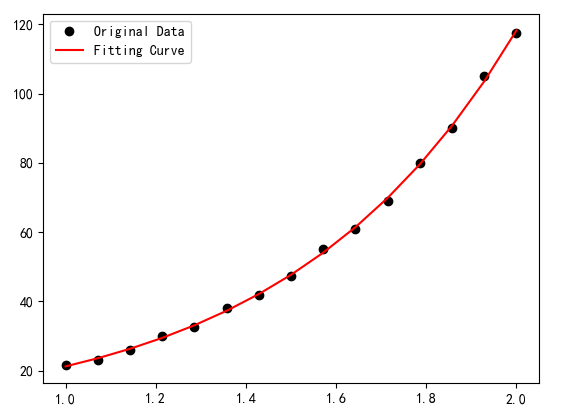从结果输出的5个参数上来看，a、b、c、d和e跟我们自己预设的函数原型中的参数是不一致的，但是不影响我们拟合得到所需要的曲线，这样的参数不一定会是唯一的，可能跟我们函数曲线拟合的时候所使用到的点数据过少有关系吧，毕竟函数本身还是比较复杂的。


展开全文• 我们都知道对数函数是以幂（真数）为自变量，指数为因变量，底数为常量的函数。对数函数是6类基本初等函数之一。对数的定义是： 如果ax =N（a>0，且a≠1），那么数x叫做以a为底N的对数，记作x=logaN，读作以a为...
我们都知道对数函数是以幂（真数）为自变量，指数为因变量，底数为常量的函数。对数函数是6类基本初等函数之一。对数的定义是：

如果ax =N（a>0，且a≠1），那么数x叫做以a为底N的对数，记作x=logaN，读作以a为底N的对数，其中a叫做对数的底数，N叫做真数。
一般地，函数y=logaX（a>0，且a≠1）叫做对数函数，也就是说以幂（真数）为自变量，指数为因变量，底数为常量的函数，叫对数函数。
其中x是自变量，函数的定义域是（0，+∞），即x>0。它实际上就是指数函数的反函数，可表示为x=ay。因此指数函数里对于a的规定，同样适用于对数函数。
但是这并没有告知学习者对数出现的历史渊源，为何要引入对数这一概念，我私以为学习一个东西必然要学习其历史以及其存在的意义才能让我们对其掌握的更加透彻。经过一系列的查询后有了些个人理解因此记录下来以便作为一个参考给有其他有相同问题的朋友。
首先我众所周知的坐标系是以1为单位的如图：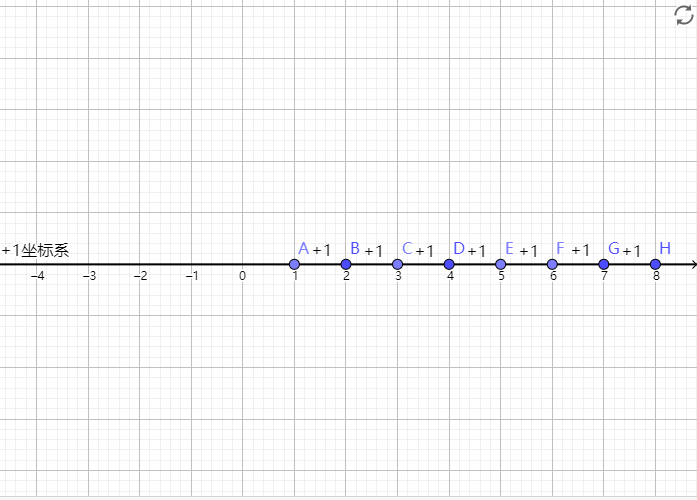但是当我们将加法轴换为乘法轴后坐标系想要继续画下去就会很难，因为指数的增长太快了。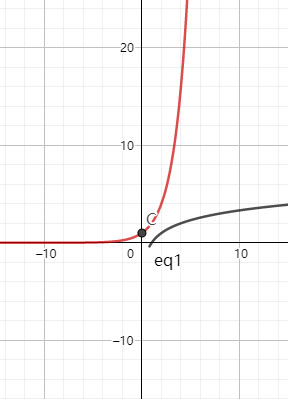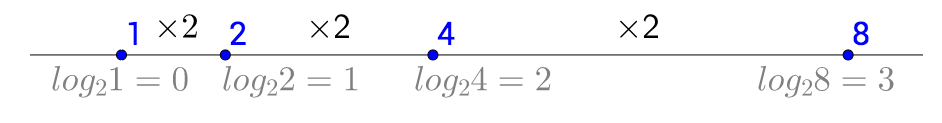但是当我们将对数当做坐标轴的横坐标并将数值等距离摆放将解决这个问题：另外对数运算中的og(a)(MN)=log(a)(M)+log(a)(N)运算将大大降低天文数字的计算时间，通过对数尺的例子就能很好体现：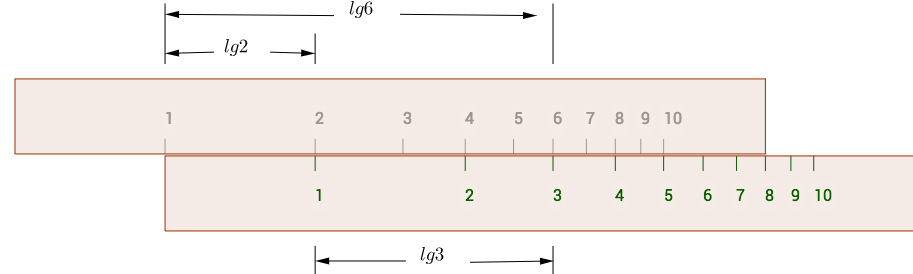展开全文数学
• 在学习机器学习相关理论时，我们常常会会在公式中遇到指数函数和对数函数，但是很时候我们并不理解这些函数的的真正作用，这里结合几个机器学习中的公式来具体分析一下指数函数和对数函数的作用 指数函数 由上图...指数函数 机器学习 softmax 深度学习
• 指数函数的性质 　先来复习一下中学的课程： 指数函数的导数 　对f(x) = ax求导： ...　函数在某一点导数的几何意义是该点处切线的斜率，所以M(a)也就是ax在x=0处切线的斜率。 　如果y=2x，...数学 指数函数
• 对于损失函数，像用平方损失函数 L(Y, f(X)) = (Y - f(X))^2或者绝对值损失函数都十分直观，但是对数损失函数 L(Y, P(Y|X)) = -log P(Y|X)是如何来度量损失的呢？ 在李航《统计学习方法第二版》11页中提到了一个...
• 自然对数是 log(x)。十为第的对数是 log10(x)。其他底的对数，用换底公式。alog(x)是单精度自然对数。dlog(x)是双精度自然对数。clog(x)是复数度自然对数。log(x)表示自然对数。扩展资料：人们用的是十进制，古人掰...
• 最大熵模型中的对数似然函数的解释 最近在学习最大熵模型，看到极大似然估计这部分，没有看明白条件概率分布p(y|x)p(y|x)p(y|x)的对数似然函数。上网查了很多资料都没有一个合理的解释。基本直接给出对数似然函数的...极大似然估计
• 3.5对数-凹函数和对数-凸函数 定义 相关性质 定义 称函数对数凹，如果是凹函数。 称函数对数凸，如果是凸函数函数f是对数凸的当且仅当1/f是对数凹的。 当时，，相当于对log(f)进行扩展值延伸，此时，如果...
• 我早期的程序就没有精度控制，结果在实现三角函数和开N次方函数时，一直不成功。 N!就是1-N的公倍数，所以能整除， 1-N的最少公倍数是多少？用最小公倍数代替 N!效果会更好 这个公式的提速原理是 精度大于N！的位长 ...大数运算
• 指数函数和自然对数我经常想e真正的意义什么呢？不是字母本身含义，而是作为一个数学常数的含义通过查阅自然对数的定义，你会发现：这个数学常数e是基于自然对数产生的。自然对数...
• 函数(乘方)｜指数(函数)｜对数(函数)｜及其运算法则2018-09-23求n个相同因数的积的运算，叫做乘方(power)，乘方的结果叫做幂(power)，在a^n(n 标在右上角)中，a叫做底数，n叫做指数，当a^n(n标在右上角)看作a的n...
• 所以数组处理函数一般都传一个数组长度，当然也可以使用数组指针，不过这么写好像就没啥意义了~ 下图: (通过数组指针 转载于:https://www.cnblogs.com/eret9616/p/10308001.html...
• 非线性函数包括：指数函数、幂函数、对数函数、多项式函数等等基本初等函数 从数学的角度如何区别于什么是线性函数，什么是非线性函数呢？ 对于一个函数y=f(x)来说，如果他是有线性的，则必须要满足两个法则： 比例...
• 在这个物欲横流的社会中，任何物品都是明码...而提到“鼋鼍”，或许我们完全不知道这是什么，但是至少我们学习到了这样一个新的词汇。因此，我们要搞清楚一件非常非常 不确定的事，或是我们一无所知的事情，就需要了算法 数学 数据挖掘
• 它仅在线性回归的基础上，在特征到结果的映射中加入了一层sigmoid函数（非线性）映射，即先把特征线性求和，然后使用sigmoid函数来预测。 sigmoid函数   当sigmoid函数作为神经元的激活函数时，有两种较好的...
• 关于对数似然法原理，网上博客众说纷纭，但能说清楚，解释对数似然公式为何如此却寥寥无几。今天我就发个博客，来和大家讨论一下。 （未经博主允许不得转载） ——————————————————...机器学习 数学原理
• 函数 、 指数 、 对数 、底数 参考文章 https://www.shuxuele.com/algebra/exponents-logarithms.html 幂函数 y=xα（α为有理数） 幂函数一般形式 x称为底数 α称为指数 幂函数在高考数学中有很大份量，在...
• 1 ，真实值函数推导 ： 真实值正态曲线 定义 ： 跟数据曲线很相似的函数 ...2 ，似然函数意义 ： 当前样本的概率 真实值函数 ： 个体概率 似然函数 ： 将样本中的每个值，带入到方程中，将每个样本的概率python
• 之所以这样做是基于对数函数在其定义域内是单调增函数，取对数后不会改变数据的相对关系，取对数作用主要有： 1. 缩小数据的绝对数值，方便计算。 例如，每个数据项的值都很大，许多这样的值进行计算可能对超过...
• 什么80%的码农都做不了架构师？>>> ...
• 目的:用于Exp(2)是e的平方，指数函数是数学中的一个重要函数。...它的反函数是自然对数ln(x)，定义在所有正数x上参考:百度百科- exp。二、EXP是什么功能 Exp，高等数学中基于自然常数e的指数函数。用途:表...
• 什么是目标函数？定义是：指所关心的目标与相关的因素的函数关系。举个例子，假如我们想要预测公司楼下手抓饼店明天能卖多少张手抓饼，已知过去10天每天卖多少，以及每天的天气情况，是否有节假日，和手抓饼店老板和...正则化
• 在Python的numpy中，对类似array=[[1,2,3],[4,...不过在此之前，先说明Python中map函数与zip(*)的使用。 一、map函数 首先Python中的map函数是很简单的。意为将第二个参数（一般是数组）中的每一个项，处理为第一个参Python map zip 数组转置 array...